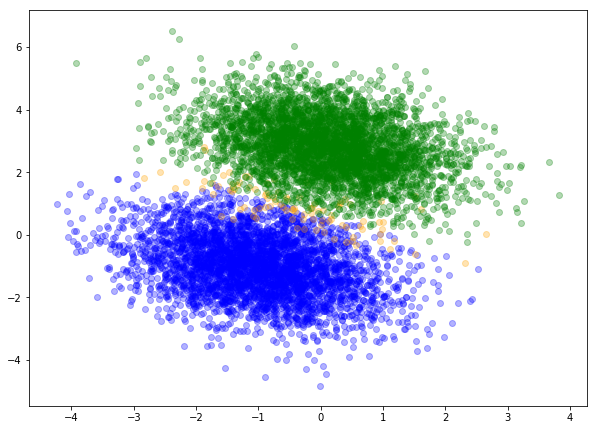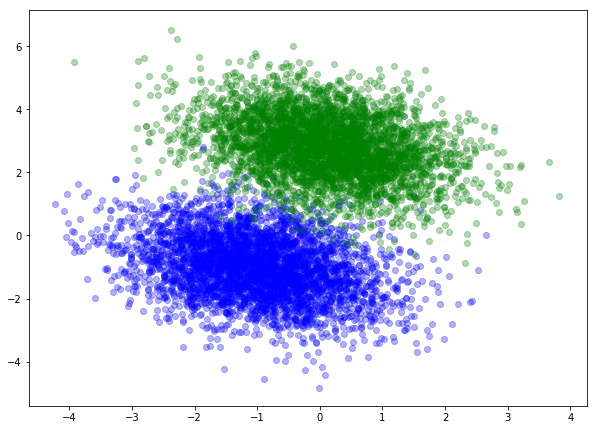# Logistic regression from scratchLogistics regression is one of the most used and successful machine learning models. Unlike linear regression, logistic regression does not have a closed-form analytical solution so we have to resort to numerical methods to solve it.

In this example we will employ batch gradient descent method for this purpose.

Our first step is generating initial set of data points.

## Data

import numpy as np
import matplotlib.pyplot as plt
%matplotlib inline

np.random.seed(1)
number_of_points=4000
learning_rate= 1e-5
iterations=200000
means=[[-1,-1],[0.1,2.8]]
cov=-0.3
covariances=[[[1,cov],[cov,1]],[[1,cov],[cov,1]]] # if changing make sure covariance matrix is positive semi definite
a=np.random.multivariate_normal(means,covariances,number_of_points)
b=np.random.multivariate_normal(means,covariances,number_of_points)

plt.figure(figsize=(10,16))
plt.subplot(2, 1, 1)
plt.scatter(a[:,0],a[:,1],c='blue',alpha=0.3)
plt.scatter(b[:,0],b[:,1],c='green',alpha=0.3)
X=np.vstack((a,b))
X=np.hstack((np.ones((X.shape,1)),X))
y=np.array([i//number_of_points for i in range(2*number_of_points)])

Our code generated two clusters of data with partial overlap:## Training

Before we start with training the model, we first need to define several functions that will help us in gradient descent search for optimal model:

def sigmoid(s):
return 1/(1+np.exp(-s))

def binary_cross_entropy(theta,X,y):
return -(1/len(y))*(y*np.log(sigmoid(np.dot(X,theta)))+(1-y)*np.log(1-sigmoid(np.dot(X,theta)))).sum()

return np.dot(X.T, (y-sigmoid(np.dot(X,theta))))

def predict(theta,X):
y_predict=[]
for t in X:
y_predict.append(1) if sigmoid(np.dot(theta,t))>0.5 else y_predict.append(0)
return y_predict

def colors(s):
if s==0:
return 'blue'
elif s==1:
return 'green'
else:
return 'orange'

def training(X,y,learning_rate):
theta=np.zeros(X.shape)
for i in range(iterations):
if i % 10000 ==0:
print("Current weights: ", theta)
print("Current cost after %d steps is: %.2f " % (i,binary_cross_entropy(theta,X,y)))
return theta



After running the training code:

theta_result=training(X,y,learning_rate)
print('Final set of weights: ',theta_result)
print('Final log loss: ',binary_cross_entropy(theta_result,X,y))
plt.figure(figsize=(10,16))
y_predict=predict(theta_result,X)
classify=[]
for i,p in enumerate(y_predict):
if y_predict[i]==y[i]:
classify.append(y_predict[i])
else:
classify.append(2)
plt.subplot(2, 1, 2)
for i,x in enumerate(X):
plt.scatter(x,x,alpha=0.3,c=colors(classify[i]))

We obtain the final set of weights and log loss:

Final set of weights:  [-2.87752162  2.48418066  4.44207557]
Final log loss:  0.03452318607035479

## Visually inspecting incorrectly classified points

To assess the accuracy in chart, we plotted all points that are incorrectly classified by the model with orange color:## Comparison to scikit-learn results

In last part of our article, we will make comparison of our results with those obtained with scikit-learn library:

#comparison with Scikit Logistic Regression
X=np.vstack((a,b))
model=LogisticRegression(C=1e4) #high C ensures cost with no regularisation
model=model.fit(X, y)
print('Final set of weights (scikitlearn)', model.intercept_, model.coef_)

The weights obtained with scikit-learn are as follows:

Final set of weights (scikitlearn) [-2.87307206] [[2.48049384 4.4352298 ]]

and only minimally deviate from the weights obtained above.